##Brocard points, Brocard angle

Below we construct the [first] Brocard point of triangle ABC. Take the orientation A- >B- >C and draw the circles {ABD} tangent at BC, {BCD} tangent to CA and {CAD} tangent to AB. They intersect at a common point D. This is the first Brocard point of ABC. Here are its basic properties:
(1) ang(DBC) = ang(DCA) = ang(DAB) = omega = [Brocard angle of the triangle].
(2) |BE|/|DE| = |AE|/|BE| = > |BE|˛ = |AE||DE|.
(3) |BD| = (c/sin(B))*sin(omega).
(4) The trilinear coordinates of D are proportional to (|BD|:|CD|:|AD|) = ((c/b):(a/c):(b/a)).
(5) cot(omega) = (|AB|˛+|BC|˛+|CA|˛)/(4area(ABC)) = cot(A)+cot(B)+cot(C).
The [second] Brocard point of the triangle ABC is defined by a similar construction: Take the reverse orientation A- >C- >B and draw circles {ABD'} tangent to AC, {ACD'} tangent to BA and {BAD'} tangent to CB. D' is the common intersection point of the three circles. Analogous formulas to (1)-(4) are valid for the second Brocard point. In particular the angle omega' is equal to omega and the trilinear coordinates of the second Brocard point are ((b/c):(c/a):(a/b)).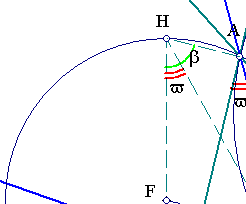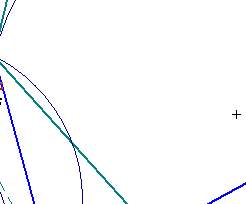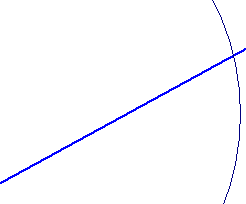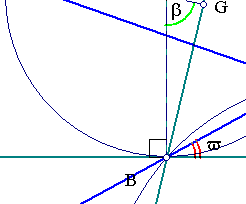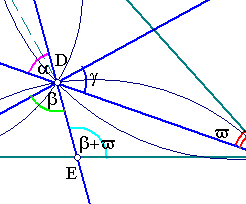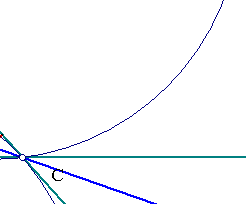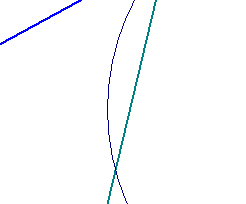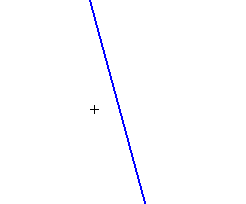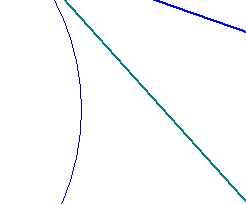A further property of the Brocard points is that they have pedal triangles similar to the original triangle ABC. This and some related figures are examined in the file BrocardPivot.html .

In the file Brocard2.html we discuss the properties of another figure, resulting right from the definition of the Brocard points.

 Produced with EucliDraw© http://users.math.uoc.gr/~pamfilos/